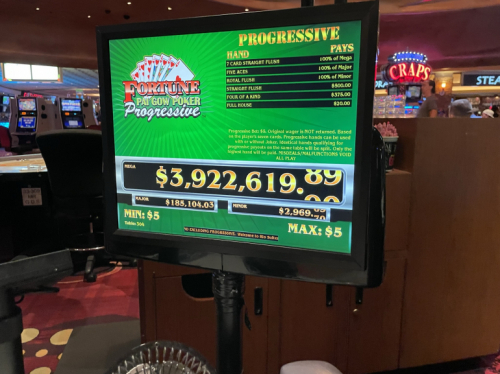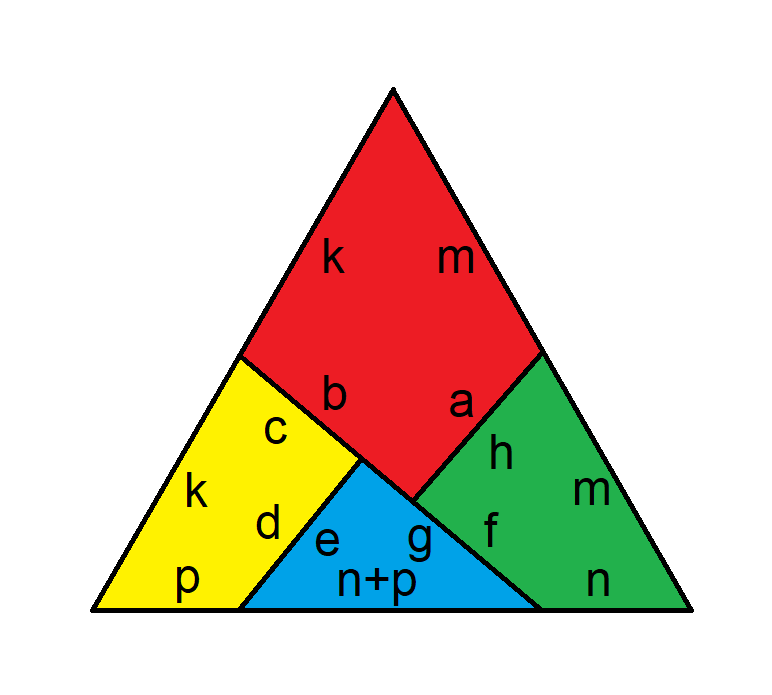I see the Face Up Pai Gow Poker Mega progressive at the Caesars properties in Las Vegas is over \$3.9 million. Is this currently a good bet?

anonymous

I checked various Caesars properties on October 1 and found this pay table for the \$5 progressive Face Up Pai Gow Poker bet.

• Seven-card straight flush = \$3,922,620
• Five aces = \$185,104
• Royal flush = \$2,969
• Straight flush = \$500
• Four of a kind = \$375
• Full house = \$20I analyze this bet in my page on Face Up Pai Gow Poker. In this case, the Mega Jackpot, for a seven-card straight flush, is unusually high. I believe it to usually be in the low five-figure range. The bottom line is this \$5 bet has an expected return of \$9.55! That means an expected profit of \$4.55.

You still have to make a negative bet on the base game. The minimum on that ranges from \$15 to \$100. At a house edge of 1.81%, here is the expected loss by bet amount on the base game.

• \$15 bet = \$0.27 loss
• \$25 bet = \$0.45 loss
• \$50 bet = \$0.90 loss
• \$100 bet = \$1.81 loss

At a base bet of \$251.61 and \$5 on the progressive, the combined game would have zero house edge.

However, two big reasons not to bet it are the 37% highest marginal federal income tax rate and the huge volatility. The average player who doesn't hit any of the top three progressives can expect to lose \$3.38 a hand at a \$15 base bet and \$3.56 at \$25. That adds up on an hourly basis.

Still, it is, mathematically, a very positive bet. At a \$15 base bet, the combined player advantage is 21.39%. You don't see that every day.

This question is asked and discussed in my forum at Wizard of Vegas.

I hear the MGM properties in Vegas now charge the vig on buy bets after a win only. How does this change the house edge?

WABJ11

I believe the already charged the vig after a win only on the 4 and 10. So, there is no effect there. Here is the house edge on the 4 and 10 all three ways you might bet them:

• Place bet (pays 9 to 5) — 6.67%
• Buy bet (commission always paid) — 4.76%
• Buy bet (commission paid on wins only) — 1.76%

On the 5 and 9, this is good news, lowing the house edge from 4.00% on a place bet to 2.00%.

• Place bet (pays 7 to 5) — 4.00%
• Buy bet (commission always paid) — 4.76%
• Buy bet (commission paid on wins only) — 2.00%

On the 6 and 8, it doesn't matter, as the house edge on place bets is still lower.

• Place bet (pays 7 to 6) — 1.52%
• Buy bet (commission always paid) — 4.76%
• Buy bet (commission paid on wins only) — 2.27%

This question is asked and discussed in my forum at Wizard of Vegas.

In episode 4 of season 37 of Survivor, there was a challenge in which four puzzles pieces could be arranged into both a square and an equilateral triangle. What would be the exact dimensions of the pieces for this to work?

anonymous

First, let's make a proper diagram and label it.Following is just the diagram of the solved triangle.Next, assuming the sides of the square are length 1, here is just the length of each letter in the diagram above.

a = d = e = h = 1/2

b = g = SQRT((4-SQRT(3))/(4*SQRT(3))) =~ 0.572145321740575

c = f = 1-SQRT((4-SQRT(3))/(4*SQRT(3))) =~ 0.427854678259425

k = m = 1/SQRT(SQRT(3)) =~ 0.759835685651592

n = (3-SQRT(4*SQRT(3)-3))/(2*SQRT(SQRT(3))) =~ 0.386767938902275

p = (SQRT(4*SQRT(3)-3)-1)/(2*SQRT(SQRT(3))) =~ 0.373067746749317

Finally, here is my full solution (PDF).

This question is asked and discussed in my forum at Wizard of Vegas.